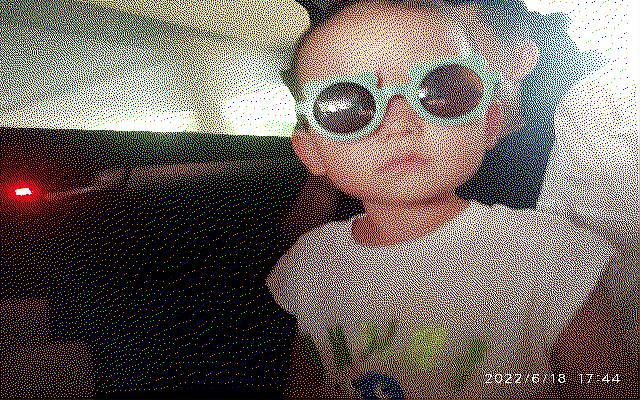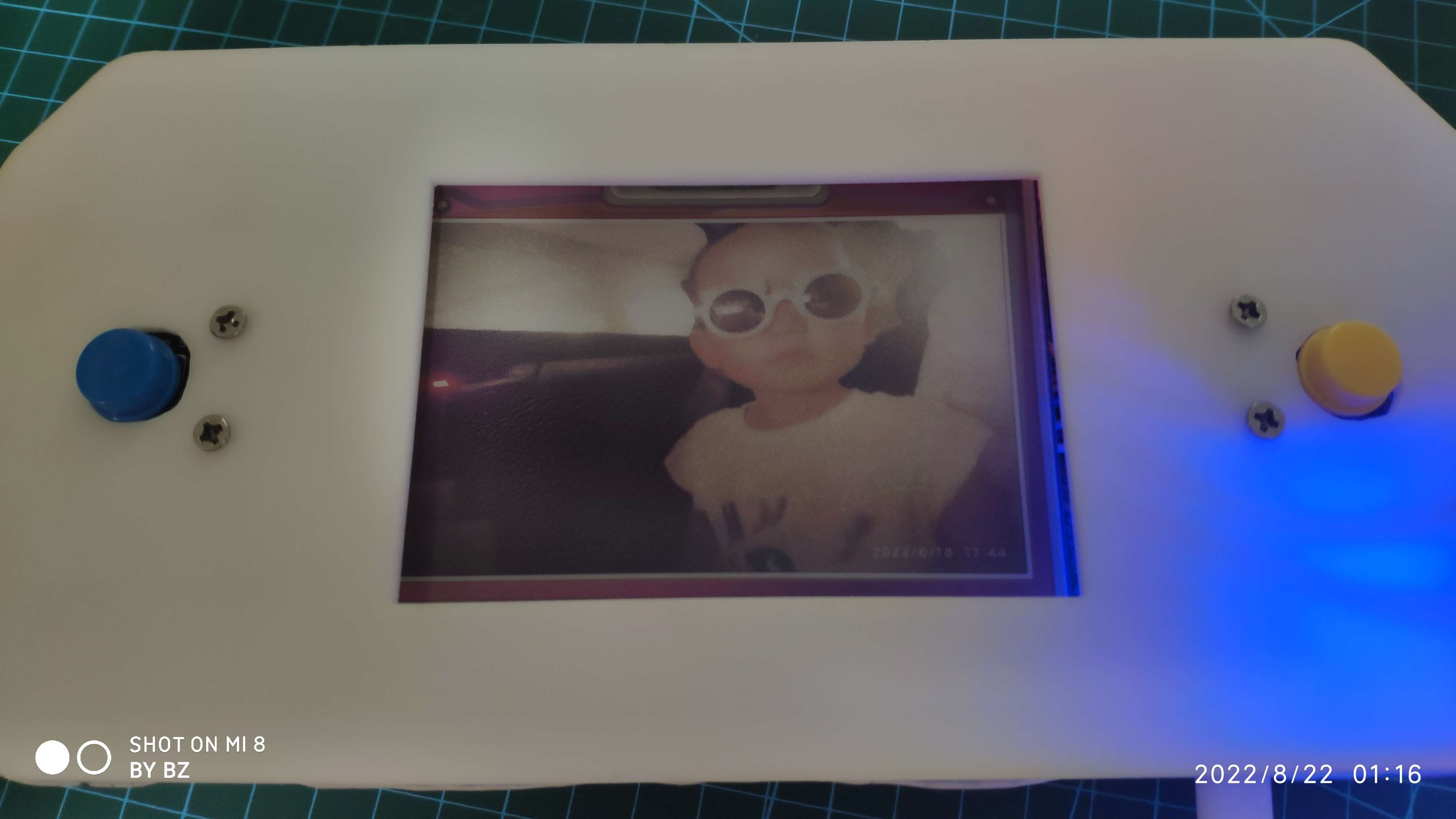## 音乐相册

  1 2 3 4 5 6 7 8 9 10 11 12 13 14 15 16 17 18 19 20 21 22 23 24 25 26 27 28 29 30 31  class Mixer_thread(threading.Thread): # 继承父类threading.Thread def __init__(self, mp3_dir): threading.Thread.__init__(self) self.mp3_paths = glob.glob(os.path.join(mp3_dir, '*.mp3')) logging.info(f"len(self.mp3_paths):{len(self.mp3_paths)}") self.index = 0 self.target_index = self.index def run(self): # 把要执行的代码写到run函数里面 线程在创建后会直接运行run函数 while True: if len(self.mp3_paths) > 1: while self.target_index == self.index: # 相等说明没有外界修改 self.target_index = random.choice(range(len(self.mp3_paths))) logging.debug(f"Mixer_thread run self.target_index:{self.target_index}") if 0 <= self.target_index < len(self.mp3_paths): # target_index有效 self.index = self.target_index logging.info(f"Mixer_thread run mp3_path:{self.mp3_paths[self.index]}") mixer.music.load(self.mp3_paths[self.index]) mixer.music.play() while self.target_index == self.index and mixer.music.get_busy(): time.sleep(0.1) else: self.index = self.target_index def pre_music(self): self.target_index = (self.index + len(self.mp3_paths) - 1) % len(self.mp3_paths) logging.debug(f"pre_music self.target_index:{self.target_index}") def next_music(self): self.target_index = (self.index + 1) % len(self.mp3_paths) logging.debug(f"next_music self.target_index:{self.target_index}") 

## 自动转换照片

### 最简单的思路：最近距离法

  1 2 3 4 5 6 7 8 9 10 11 12 13 14 15 16  def _get_color_distance(color_a, color_b): return (color_a - color_b) ** 2 + (color_a - color_b) ** 2 + (color_a - color_b) ** 2 def _get_closest_color(color): min_distance = 255 ** 2 + 255 ** 2 + 255 ** 2 + 1 closest_color = color_act try: for color_act_ in color_act: distance = _get_color_distance(color_act_, color) if distance < min_distance: min_distance = distance closest_color = color_act_ except: traceback.print_exc() return closest_color### 色彩均衡

 1 2  image = ImageEnhance.Color(image) image = image.enhance(factor=1.5)### Floyd-Steinberg扩散抖动算法

  1 2 3 4 5 6 7 8 9 10 11 12 13 14 15 16 17 18 19 20 21 22 23 24 25 26 27 28 29 30 31 32 33 34 35 36 37 38 39 40 41 42 43 44 45 46 47  def floyd_steinberg_dither(img): pixel = img.load() x_lim, y_lim = img.size for y in range(1, y_lim): for x in range(1, x_lim): red_oldpixel, green_oldpixel, blue_oldpixel = pixel[x, y] print(f"floyd_steinberg_dither : old pixel[x, y]: {pixel[x, y]}") print(f"floyd_steinberg_dither : _get_closest_color(pixel[x, y]): {_get_closest_color(pixel[x, y])}") pixel[x, y] = tuple(_get_closest_color(pixel[x, y])) red_newpixel, green_newpixel, blue_newpixel = pixel[x, y] red_error = red_oldpixel - red_newpixel green_error = green_oldpixel - green_newpixel blue_error = blue_oldpixel - blue_newpixel if x < x_lim - 1: red = pixel[x + 1, y] + round(red_error * 7 / 16) green = pixel[x + 1, y] + round(green_error * 7 / 16) blue = pixel[x + 1, y] + round(blue_error * 7 / 16) pixel[x + 1, y] = (red, green, blue) if x > 1 and y < y_lim - 1: red = pixel[x - 1, y + 1] + round(red_error * 3 / 16) green = pixel[x - 1, y + 1] + round(green_error * 3 / 16) blue = pixel[x - 1, y + 1] + round(blue_error * 3 / 16) pixel[x - 1, y + 1] = (red, green, blue) if y < y_lim - 1: red = pixel[x, y + 1] + round(red_error * 5 / 16) green = pixel[x, y + 1] + round(green_error * 5 / 16) blue = pixel[x, y + 1] + round(blue_error * 5 / 16) pixel[x, y + 1] = (red, green, blue) if x < x_lim - 1 and y < y_lim - 1: red = pixel[x + 1, y + 1] + round(red_error * 1 / 16) green = pixel[x + 1, y + 1] + round(green_error * 1 / 16) blue = pixel[x + 1, y + 1] + round(blue_error * 1 / 16) pixel[x + 1, y + 1] = (red, green, blue) return img 

Floyd-Steinberg扩散抖动算法：## 缓存